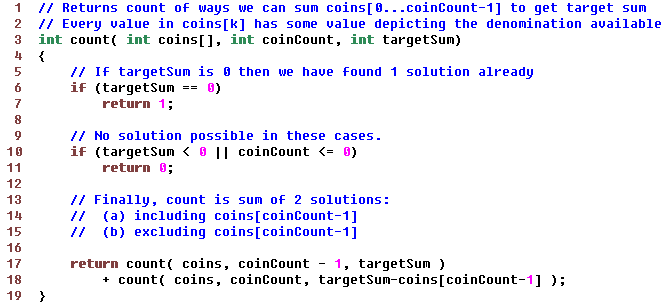Data Structuresand Algorithms Good OldJava AdvancedInterview Topics Cloud andDatabases Web Designand Development Must-knowTools Good ToKnow

## Coins

Given an infinite supply of ‘m’ coin denominations S[m] = {S1, S2... Sm}, calculate all the different combinations which can be used to get change for some quantity ‘N’

So, if N = 4 and S = {1,2,3}, then different ways possible are

{1,1,1,1}, {2,1,1}, {3,1}, {2,2}

This can be solved recursively by considering 2 cases in every step.

One case contains coin m and one case does not contain coin m.It’s clear that the above can be optimized using dynamic programming because we are calculating lower functions repeatedly.

We can build a table for all the lower values of targetSum and coins and then derive higher column values from them.

This can be done as follows:The above has a time complexity of O(targetSum * coinCount) and a space complexity of the same order.

But if we look closely, we don't really care so much about those table entries where coinCount is less than the given coinCount. Those columns are just being used to help the calculation.

We should be able to just use a single column from the above table.

This can be done by considering a single coin at a time and adding the number of cases possible by its usage to the cases where it was not used.

Java Code Sample Execution
```public class MaxWaysToMakeSum
{
public static void main(String[] args)
{
maxWays(10, new int[]{1,2});
maxWays(10, new int[]{1,2,3});
maxWays(10, new int[]{1,3,5});
maxWays(10, new int[]{1,4,7});
}

static void maxWays (int targetSum, int coins[])
{
debugInput(targetSum, coins);
// Lookup to store solutions for all sums from
// 0 to targetSum.
int sums[] = new int [targetSum+1];

// Used to indicate that a solution exists when
// a coin equal to current sum is used.
// i.e. sums[sumK-coin] should return 1 when sumK equals coin
sums = 1;

// Pick all coins one by one and update the lookup 'sums'
// to indicate the effect of introducing that coin
for (int coin: coins)
{
// For sums less than current coin value, there would be
// no effect of introducing this coin, hence we begin from
// the sum atleast equal in value to current coin
for (int sumK=coin; sumK<=targetSum; sumK++)
sums[sumK] =
sums[sumK] + // current coin is not used
sums[sumK-coin]; // current coin is used
print(sums);
}
System.out.println("Maximum no of ways = " + sums[targetSum]);
}

/********************* DEBUG FUNCTIONS **********************/
private static void debugInput(int targetSum, int[] coins)
{
System.out.println ("\n\nTargetSum = " + targetSum +
", Coins =" + arrayToString(coins));
System.out.print(" ");
for (int i=0; i<=targetSum; i++)
System.out.print(String.format("[%2d]", i) + " ");
System.out.println();
}
private static void print(int[] s)
{
System.out.println (arrayToString(s));
}
private static String arrayToString(int[] sums)
{
String buf = "";
for (int s: sums)
buf += String.format("%4d", s) + " ";
return buf;
}
}
```

Below output for each execution is
1. Numbers in square brackets stand for the
"sums" lookup array
2. Every row after that lists the max no. of
ways for each sum using coink

```TargetSum = 10, Coins = {1,2}
SUMS =  [ 0] [ 1] [ 2] [ 3] [ 4] [ 5] [ 6] [ 7] [ 8] [ 9] 
Coin(1)   1    1    1    1    1    1    1    1    1    1    1
Coin(2)   1    1    2    2    3    3    4    4    5    5    6
Maximum no of ways = 6

TargetSum = 10, Coins = {1, 2, 3}
SUMS =  [ 0] [ 1] [ 2] [ 3] [ 4] [ 5] [ 6] [ 7] [ 8] [ 9] 
Coin(1)   1    1    1    1    1    1    1    1    1    1    1
Coin(2)   1    1    2    2    3    3    4    4    5    5    6
Coin(3)   1    1    2    3    4    5    7    8   10   12   14
Maximum no of ways = 14

TargetSum = 10, Coins = {1, 3, 5}
SUMS =  [ 0] [ 1] [ 2] [ 3] [ 4] [ 5] [ 6] [ 7] [ 8] [ 9] 
Coin(1)   1    1    1    1    1    1    1    1    1    1    1
Coin(3)   1    1    1    2    2    2    3    3    3    4    4
Coin(5)   1    1    1    2    2    3    4    4    5    6    7
Maximum no of ways = 7

TargetSum = 10, Coins = {1, 4, 7}
SUMS =  [ 0] [ 1] [ 2] [ 3] [ 4] [ 5] [ 6] [ 7] [ 8] [ 9] 
Coin(1)   1    1    1    1    1    1    1    1    1    1    1
Coin(4)   1    1    1    1    2    2    2    2    3    3    3
Coin(7)   1    1    1    1    2    2    2    3    4    4    4
Maximum no of ways = 4
```

Like us on Facebook to remain in touch
with the latest in technology and tutorials!

Got a thought to share or found a
bug in the code?
We'd love to hear from you:

 Name: Email: (Your email is not shared with anybody) Comment: